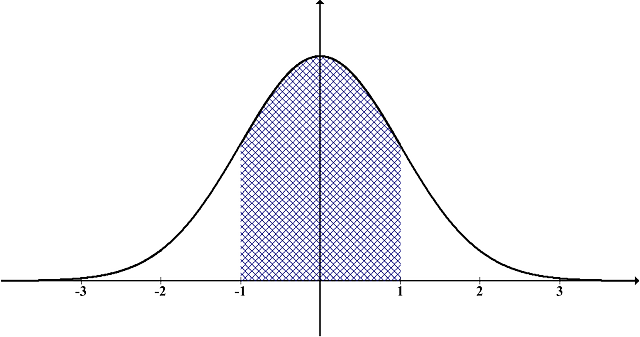# Z-test for One Population Mean

Instructions: This calculator conducts a Z-test for one population mean ($$\mu$$), with known population standard deviation ($$\sigma$$). Please select the null and alternative hypotheses, type the hypothesized mean, the significance level, the sample mean, the population standard deviation, and the sample size, and the results of the z-test will be displayed for you:

Ho: $$\mu$$ $$\mu_0$$
Ha: $$\mu$$ $$\mu_0$$Hypothesized Mean ($$\mu_0$$)Sample Mean ($$\bar X$$)Population St. Dev. ($$\sigma$$)Sample Size (n)Significance Level ($$\alpha$$)

## How to Conduct a Z-Test for One Population Mean?

More about the z-test for one mean so you can better interpret the results obtained by this solver: A z-test for one mean is a hypothesis test that attempts to make a claim about the population mean ($$\mu$$).

The test has two non-overlapping hypotheses, the null and the alternative hypothesis. The null hypothesis is a statement about the population mean, under the assumption of no effect, and the alternative hypothesis is the complementary hypothesis to the null hypothesis. The main properties of a one sample z-test for one population mean are:

• Depending on our knowledge about the "no effect" situation, the z-test can be two-tailed, left-tailed or right-tailed

• The main principle of hypothesis testing is that the null hypothesis is rejected if the test statistic obtained is sufficiently unlikely under the assumption that the null hypothesis is true

• The p-value is the probability of obtaining sample results as extreme or more extreme than the sample results obtained, under the assumption that the null hypothesis is true

• In a hypothesis tests there are two types of errors. Type I error occurs when we reject a true null hypothesis, and the Type II error occurs when we fail to reject a false null hypothesis

### Uses of this z-test calculator

What can you do with this z-test statistic calculator for hypothesis testing? The formula for a z-statistic is

$z = \frac{\bar X - \mu_0}{\sigma/\sqrt{n}}$

The null hypothesis is rejected when the z-statistic lies on the rejection region, which is determined by the significance level ($$\alpha$$) and the type of tail (two-tailed, left-tailed or right-tailed).

### What if the population standard deviation is not known?

It frequently happens that you don't actually know the population standard deviation, in which case you need to use a t-test for one mean calculator instead, which adjusts for that by using the sample standard deviation, by using a slightly different distribution (the t-distribution)

### How to calculate p-value in the context of a z-test?

The answer depends on whether you are using a two-tailed, a left-tailed or a right-tailed test. Say you have the calculated z-statistic, $$Z_{obs}$$.

• For a two-tailed test, the p-value is computed as: $$p = \Pr( Z > |Z_{obs}|)$$
• For a left-tailed test, the p-value is computed as: $$p = \Pr( Z < Z_{obs})$$
• For a right-tailed test, the p-value is computed as: $$p = \Pr( Z > Z_{obs})$$

where $$Z$$ has a standard normal distribution.

### Other types of Z-calculators

In case that you need to compare two population means, when you know the corresponding population standard deviations, you need to use this z-test for two means with known population standard deviations instead.

### Outlier Detection

Don't forget to detect outliers before running a z-test for one mean. It is important that outliers are detected and removed before conducting the test, but the results of the test statistics may be slanted.### Example: Application of the Z-test calculator

Question: Assume that you want to test whether or not the population mean is 12.3. You collect a representative random sample of size n = 16, and you find that the sample mean is 11.3. Also, you know that the population is 2.3. Do the sample data provide enough evidence to reject the claim that the population mean is 12.3? Use a two-tailed test, with a significance level of 0.01.

Solution:

The following information has been provided:

 Hypothesized Population Mean $$(\mu)$$ = $$12.3$$ Population Standard Deviation $$(\sigma)$$ = $$2.3$$ Sample Size $$(n)$$ = $$16$$ Sample Mean $$(\bar X)$$ = $$11.3$$ Significance Level $$(\alpha)$$ $$0.01$$

(1) Null and Alternative Hypotheses

The following null and alternative hypotheses need to be tested:

$\begin{array}{ccl} H_0: \mu & = & 12.3 \\\\ \\\\ H_a: \mu & \ne & 12.3 \end{array}$

This corresponds to a two-tailed test, for which a z-test for one mean, with known population standard deviation will be used.

(2) Rejection Region

Based on the information provided, the significance level is $$\alpha = 0.01$$, and the critical value for a two-tailed test is $$z_c = 2.58$$.

The rejection region for this two-tailed test is $$R = \{z: |z| > 2.576\}$$

(3) Test Statistics

The z-statistic is computed as follows:

$\begin{array}{ccl} z & = & \displaystyle \frac{\bar X - \mu_0}{\sigma/\sqrt{n}} \\\\ \\\\ & = & \displaystyle \frac{ 11.3 - 12.3}{ 2.3/\sqrt{ 16}} \\\\ \\\\ & = & -1.739 \end{array}$

(4) Decision about the null hypothesis

Since it is observed that $$|z| = 1.739 \le z_c = 2.576$$, it is then concluded that the null hypothesis is not rejected.

Using the P-value approach: The p-value is $$p = 0.082$$, and since $$p = 0.082 \ge 0.01$$, it is concluded that the null hypothesis is not rejected.

(5) Conclusion

It is concluded that the null hypothesis Ho is not rejected. Therefore, there is not enough evidence to claim that the population mean $$\mu$$ is different than 12.3, at the $$\alpha = 0.01$$ significance level.

Confidence Interval

The 99% confidence interval is $$9.819 < \mu < 12.781$$.